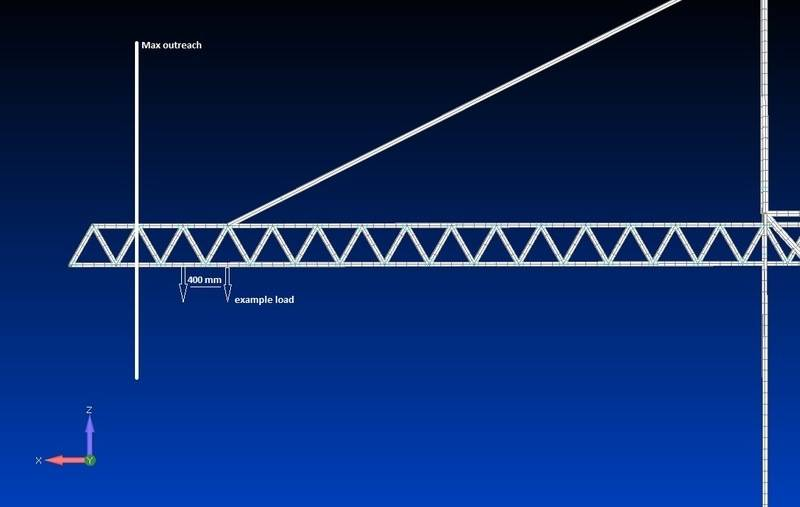# Worst load cases lattice structure

DaanD
Introduction
Dear all,
I'm working on an assignment to model a ship-to-shore crane for a FEM design course. Having modeled the crane, I now need to apply the load of the trolley (which is hoisting the container) on the boom (which in my case is a lattice structure made up of beam elements).

The total load of the trolley will be applied on the boom in 4 points (1 for each corner of the trolley) the load on an arbitrary position is shown in the image.The question
Instead of applying the load at every possible position (which are a lot), I want to find the worst load cases along the lattice structure and only calculate the stresses when the loads are in those positions. I however have a hard time determining where that might be. If the boom would be model as just a single beam, it would be a lot easier. However, thanks to the lattice structure I have a hard time imaging where to apply the loads. Perhaps someone can point me in the right direction?

## Answers and Replies

2021 Award
I think you first need to model the load at each lattice point along the boom.
Plot a curve to show where the most critical points along the boom may be.
Then model say eight smaller step positions along the boom between two lattice points in that region.

Remember that the horizontal wind load on a lattice structure can often be more than the vertical gravitational forces.

Homework Helper
If the boom would be model as just a single beam, it would be a lot easier.

You can model it approximately as a simple beam. It acts like an I-beam with the top and bottom rails as the flanges, and other parts carrying the shear load between them like the web of an I-beam.

You could calculate the equivalent "I" value approximately, by ignoring the "web" and just including the top and bottom rails. Or, take your existing model, delete the irrelevant parts, load it like a cantilever, and find the equivalent "EI" value from the deflection you get. If you have time, it would be instructive to do both and compare them (and compare them with your detailed model).

I real life you would probably design the crane by doing this backwards: start by assuming the boom is a simple beam, then figure out the lightest/cheapest way to make that beam from a truss structure.

Actually, you can probably get some useful information from shear force and bending moment diagrams, without calculating the equivalent beam properties at all - just by realizing that the structure acts like a beam.

Last edited:
Staff Emeritus